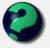# Math Is Fun Forum

Discussion about math, puzzles, games and fun.   Useful symbols: ÷ × ½ √ ∞ ≠ ≤ ≥ ≈ ⇒ ± ∈ Δ θ ∴ ∑ ∫  π  -¹ ² ³ °

You are not logged in.

## #1 2008-03-24 08:45:57

Zenith
Member
Registered: 2008-03-24
Posts: 1

### Calculate a point along a line

I have tried to solve this problem for a while now, and I always seem to get the wrong answer. I really need help.

Two points form a line. How do I find the the x and y coordinates of a point that is n units from one point going towards the other point along the line?

Diagram:

n
_________________________
A              B                          C

How do you find where B is? I turn the line into a triangle and use similar edges of it to find the answer. However, this do what I need; I want to use some sort of equation/rule to find the location of B.

Offline

## #2 2008-03-24 09:33:57

mathsyperson
ModeratorRegistered: 2005-06-22
Posts: 4,900

### Re: Calculate a point along a line

Let's say that point A is (a,b) and point C is (c,d).

To get from A to C, you need to go (c-a) units to the right, and (d-b) units up.
So the equation of the line that contains points A and C is (a,b) + t(c-a,d-b), where t is some parameter. (This is because every point on the line can be made by starting at point A and then moving some distance parallel to the line AC.)

You can also find the length AC by Pythagoras: AC = √[(c-a)²+(d-b)²].
By introducing a new variable L and saying that t = L/AC, you get the equation of the line joining A and C to be (a,b) + L/AC(c-a,d-b). This time, L indicates the distance from point A (in the direction of C).

(Hopefully I've explained that well enough. Feel free to post again if I've been unclear.)

Why did the vector cross the road?
It wanted to be normal.

Offline

## #3 2013-03-05 09:31:07

mikkel
Guest

### Re: Calculate a point along a line

That was extremely helpful for my game project I am doing, so glad I found this explanation thank you!!:D

## #4 2013-06-02 12:09:24

mathsfailure
Guest

### Re: Calculate a point along a line

(x1,y1) + t(x2-x1,y2-y1)

AC = √[(x2-x1)²+(y2-y1)²]

t = L/AC

(x1,y1) + (L/√[(x2-x1)²+(y2-y1)²])(x2-x1,y2-y1)

I don't understand how to add to (x1,y1). (a,b) + 1 = ???
I don't understand how to extract a result after substituting L for a distance.

Let me try it anyway.
(x1,y1) = (10, 20)
(x2,y2) = (20, 40)
L = 5

x2-x1 = 10
y2-y1 = 20

AC = √(100 + 400) = 22.4
t = 5/22.4 = 0.223

(10, 20) + 0.223(10, 20)

I'm not sure what to do here but I'll assume I separate the x and y values like this:

x = 10 + (0.223 * 10)
y = 20 + (0.223 * 20)

(12.2, 24.5)

## #5 2013-06-02 18:44:16

BobRegistered: 2010-06-20
Posts: 9,865

### Re: Calculate a point along a line

hi mathsfailure

Welcome to the forum.

The distance between two points is given by your equation for AC

http://www.mathsisfun.com/algebra/dista … oints.html

Your calculation for AC is correct.

The formula

enables you to work out how far along the line to go.

Your calculation of t is correct.

Yes, it is correct to deal with the x coordinates and the y coordinates separately like that.So, ..... not so much of a failure after all.Bob

Children are not defined by school ...........The Fonz
You cannot teach a man anything;  you can only help him find it within himself..........Galileo Galilei
Sometimes I deliberately make mistakes, just to test you!  …………….BobOffline